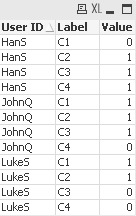# QlikView App Dev

Discussion Board for collaboration related to QlikView App Development.

Announcements
cancel
Showing results for
Did you mean:Creator

## if statement on multiple columns return all values

C1C2C3C4
1100
0111
1110

This is probably really simple, but I'm having a brain freeze on how to accomplish it. I need a logic statement that returns all of the values of 1. If I use an IF statement, the first if knocks out the subsequent values

This statement

if(C1=1,1,0,if(C2=1,1,0,if(C3=1,1,0,if(C4=1,1,0)

returns

count of C1 = 2

count of C2 = 1

count of C3 = 0

count of C4 = 0

What can I use to get the counts to accurately reflect

count of C1 = 2

count of C2 = 3

count of C3 = 2

count of C4 =1

Thanks!

10 RepliesContributor III

Hi Lawrence,

It is exactly the same thing.

The CROSSTABLE Load keeps the first column as is, and pivots the following columns.

So if I have the following table:

User IDC1C2C3C4
LukeS1100
JohnQ1110
HanS0111

And I apply the same CROSSTABLE Load I will have the following table in QlikView:And I can create the same chart and have the same results as shown before.

For example, if in addition to "User ID" I also want to have "Region" I use the following load example:

```Table:
CROSSTABLE(Label,Value, 2)
[User ID],
Region,
C1,
C2,
C3,
C4

FROM

(ooxml, embedded labels, table is EVENTDATE);
```

That additional parameter (the number 2) at the end of CROSSTABLE defines how many columns are not to be pivoted.  By default is 1, the first one.  By defining it as 2, I am telling it not to pivot "User ID" nor "Region".

You can escalate that as you require.

Attached is an example with User ID.